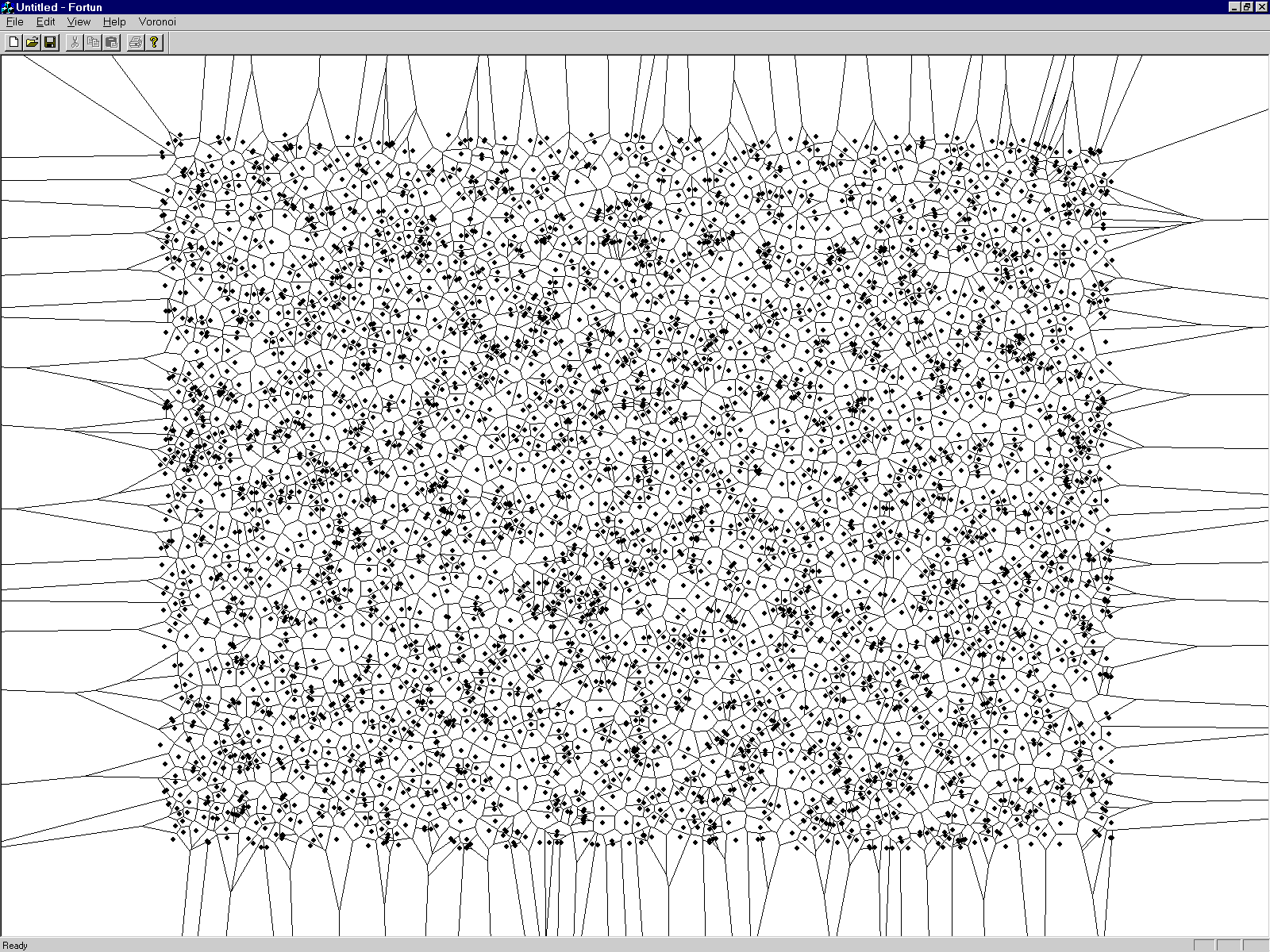## 4. Conclusions and future work

It is known from literature, the Fortune's algorithm works in O(n log2 n) time and that it is one of the simplest algorithms for constructing the Voronoi diagrams. Table 1 shows the obtained measurements of spent CPU time for different number of points. It can be concluded that the theoretical time complexity estimation is achieved also in practice. The algorithm is implemented in C++ and the measurements have been done on PC Pentium 133 MHz and 64 Mbytes RAM. The results of construction can be seen in Figure 11.

Table 1. Measurements of spent CPU time
No. of points 2000 4000 6000 8000 10000
Spent CPU time [s] 3.86 7.61 11.73 16.84 22.31Figure 11: Voronoi diagram constructed on 4000 points

Roman Cuk - roman.cuk@uni-mb.si